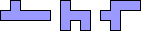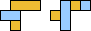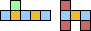# Polyomino Oddities

A polyomino oddity is a symmetrical figure made of an odd number of copies of a polyomino. For some early work on oddities, see Livio Zucca's oddities chart and Torsten Sillke's notes.

Here are minimal known oddities for asymmetrical polyominoes of orders 4 through 7. Mike Reid contributed many original and improved solutions to this catalogue, and Roger Phillips improved one of Mike's.

## Tetrominoes### Holeless Variant## Pentominoes### Holeless VariantsFor other symmetries, see Pentomino Oddities.

## Hexominoes### ImpossibleProofs.

Lemma. If a hexomino figure has rotary symmetry around a cell, the cell must be vacant. Proof. A figure made of hexominoes has an even number of cells. A figure with rotary symmetry around an occupied cell has an odd number of cells.Color the plane in red and green checks. Each tile shown has |R−G|=2. A figure with an odd number of such tiles must also have R≠G. But a figure with lateral symmetry around edges must have R=G. The same is true of rotary symmetry around an edge.Color the plane in yellow and blue stripes. Each tile shown has an odd number of cells of each color. A figure with an odd number of such tiles must also have odd numbers of yellow and blue cells. But a figure with lateral symmetry around cells must have an even number of cells of the color not in the middle. The same argument applies to rotary symmetry around a vacant cell.

For rotary symmetry around a vertex, each tile shown has Y=B in one orientation and Y≠B in the other. If a figure has an odd number of tiles, it must have an odd number of tiles in one orientation or the other. Color the plane so that the orientation with an odd number of tiles has Y≠B. Then the figure has Y≠B. But a figure with rotary symmetry around a vertex must have Y=B.In each orientation the tile shown has 4 cells of one color and 2 of the other. A figure with an odd number of such tiles must have Y≠B. But a figure with rotary symmetry around a vertex must have Y=B.Color the plane in four colors as shown. The tile shown has an odd number of cells of each color. A figure with an odd number of such tiles must also have an odd number of cells of each color. But a figure with lateral symmetry around cells must have an even number of cells of the colors not in the middle, and a figure with rotary symmetry around a vacant cell must have an even number of cells of each color.For each tile |R−Y| = |B−G| = 2. A figure with an odd number of such tiles must also have R ≠ Y and B ≠ G. But a figure with diagonal symmetry must have an equal number of cells of the two colors not on the diagonal axis.Color the plane as shown. The tile shown has an odd number of cells of each color. A figure with an odd number of such tiles must also have an odd number of cells of each color. But if a figure has diagonal symmetry, we may assume without loss of generality that all the cells on its axis are orange. Then by symmetry the number of purple cells is even.

### Other Symmetries

For other symmetries, see Hexomino Oddities.

## Heptominoes

### Trivial### Non-Trivial### Other Symmetries

For other symmetries, see Heptomino Oddities.

Last revised 2016-02-20.

Back to Polyform Oddities < Polyform Curiosities
Col. George Sicherman [ HOME | MAIL ]